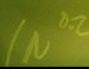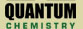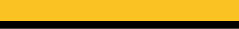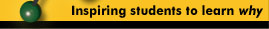Welcome to the Quantum Equation Balancing Tutor! Please type your unbalanced chemical equation in the box and click "OK", or select an equation from the menu and click "OK". This Equation Balancing Tutor is designed for beginning and novice studentswho require a higher level of guidance. This Tutor provides expert modelingof appropriate and necessary concepts by leading the student through the typesof questions that should be asked about Equation Balancing and chemistry in general.This is a demonstration version of the Equation Balancing Tutorthat only accepts equations containing carbon. The full-featured versionof the Tutor accepts any chemical equation.

 Enter an Equation or Choose an Equation H2 + O2 -> H2O C + Fe2O3 -> Fe + CO CrCl3 + AgNO3 -> Cr(NO3)3 + AgCl SO2 + O2 -> SO3 MnO2 + CO -> Mn2O3 + CO2 Mg3N2 + H2O -> Mg(OH)2 + NH3 C3H5(NO3)3 -> CO2 + H2O + N2 + O2 Cu(NO3)2 -> CuO + NO2 + O2 HCN + O2 -> N2 + CO2 + H2O C6H14 + O2 -> CO2 + H2O MnO2 + HCl -> Cl2 + MnCl2 + H2O NaCl + H2SO4 + MnO2 -> MnSO4 + Na2SO4 + H2O + Cl2